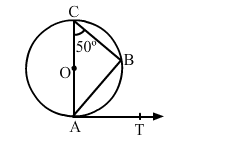# In the given figure, O is the centre of a circle. AOC is its diameter, such that ∠ACB = 50°.`
Question:

In the given figure, O is the centre of a circle. AOC is its diameter, such that ∠ACB = 50°. If AT is the tangent to the circle at the point A, then ∠BAT = ?(a) 40°
(b) 50°
(c) 60°
(d) 65°

Solution:

(b) 50°

$\angle A B C=90^{\circ}$ (A ngle in a semicircle)

In $\triangle A B C$, we have : $\angle A C B+\angle C A B+\angle A B C=180^{\circ}$

$\Rightarrow 50^{0}+\angle C A B+90^{0}=180^{\circ}$

$\Rightarrow \angle C A B=\left(180^{\circ}-140^{0}\right)$

$\Rightarrow \angle C A B=40^{\circ}$

Now, $\angle C A T=90^{\circ}$ (T angents drawn from an external point are perpendicular

to the radius at the point of contact)

$\therefore \angle C A B+\angle B A T=90^{\circ}$

$\Rightarrow 40^{\circ}+\angle B A T=90^{\circ}$

$\Rightarrow \angle B A T=\left(90^{\circ}-40^{\circ}\right)$

$\Rightarrow \angle B A T=50^{0}$